Question

# 9. When sunlight from air enters glass, the color of light that refracts the least is...

9. When sunlight from air enters glass, the color of light that refracts the least is

A. red

B. orange

C. green

D. violet

E. all refract the same amount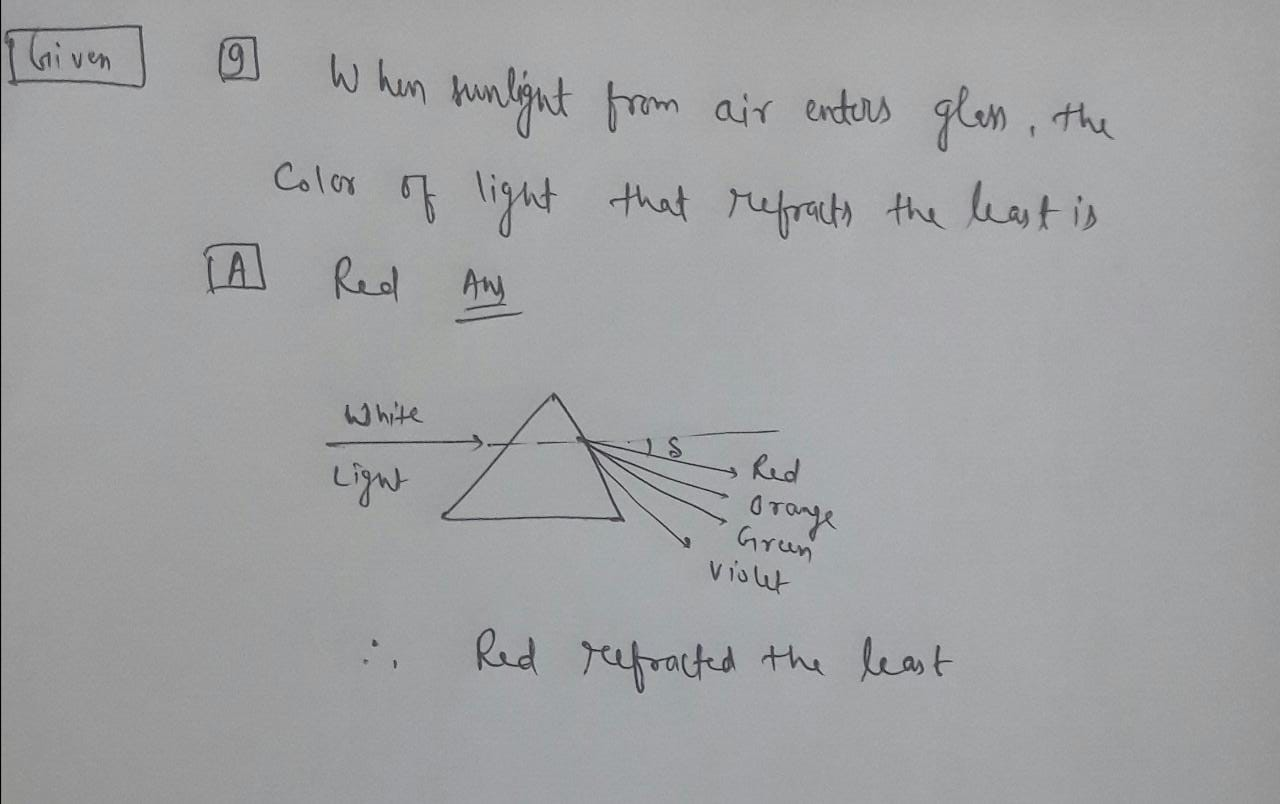#### Earn Coins

Coins can be redeemed for fabulous gifts.

Similar Homework Help Questions
• ### Which color of light undergoes the greatest refraction whenpassingfrom air to glass? Question 24 answers A....

Which color of light undergoes the greatest refraction whenpassingfrom air to glass? Question 24 answers A. red B. violet C. green D. yellow

• ### Which of the following is correct? (a) When passing through air, blue light travels slower than...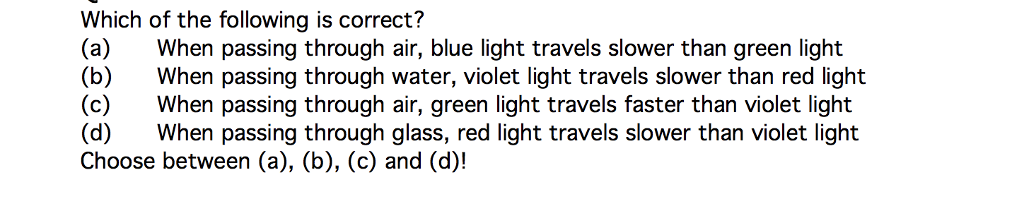Which of the following is correct? (a) When passing through air, blue light travels slower than green light (b) When passing through water, violet light travels slower than red light (c)When passing through air, green light travels faster than violet light (d) When passing through glass, red light travels slower than violet light Choose between (a), (b), (e) and (d)!

• ### 9. A ray of violet light is exactly horizontal after entering a 45-45-90° glass prism from...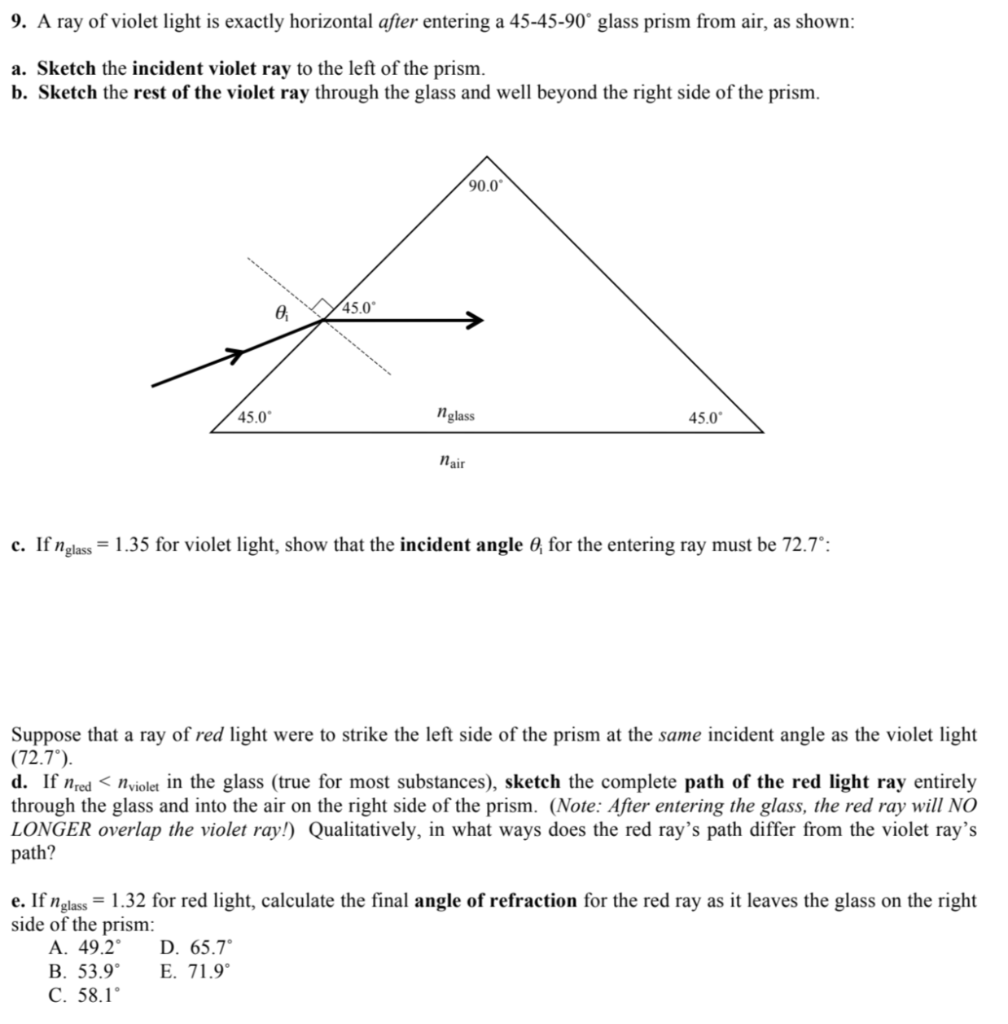9. A ray of violet light is exactly horizontal after entering a 45-45-90° glass prism from air, as shown: a. Sketch the incident violet ray to the left of the prism. b. Sketch the rest of the violet ray through the glass and well beyond the right side of the prism. 90.0 o 45.0 n glass 45.0 Nair c. If n glass = 1.35 for violet light, show that the incident angle 0, for the entering ray must be 72.7°:...

• ### A beam of light containing red (660 nm) and violet (410 nm) wavelengths travels from air,...

A beam of light containing red (660 nm) and violet (410 nm) wavelengths travels from air, through a flat piece of crown glass 1.06 cm thick, and then back to air.(a) If the beam has an angle of incidence of 20.8° in air, determine the angle at which the two colors of light emerge from the crown glass. The index of refraction respectively for red and violet light in crown glass is 1.512 and 1.530. (Enter a number to three...

• ### A ray of light passes from air (n = 1.00) into a block with a refractive...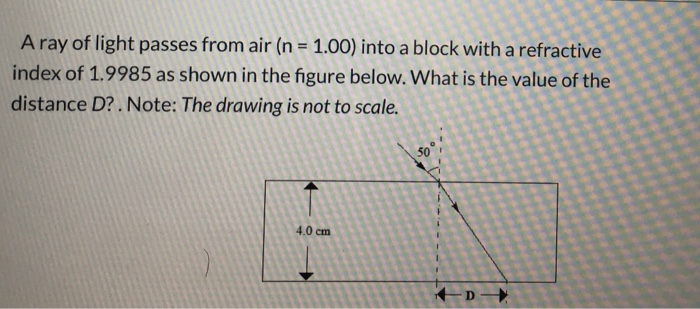A ray of light passes from air (n = 1.00) into a block with a refractive index of 1.9985 as shown in the figure below. What is the value of the distance D?. Note: The drawing is not to scale. 50 4.0 cm D- A beam of light that consists of a mixture of red (n = 1.30), green (n=1.42) and violet ( n = 1.46) light strikes a prism (surrounded by air) as shown below. An observer is located...

• ### 8. A ray of light enters a 45-45-90° glass prism from air at an angle of...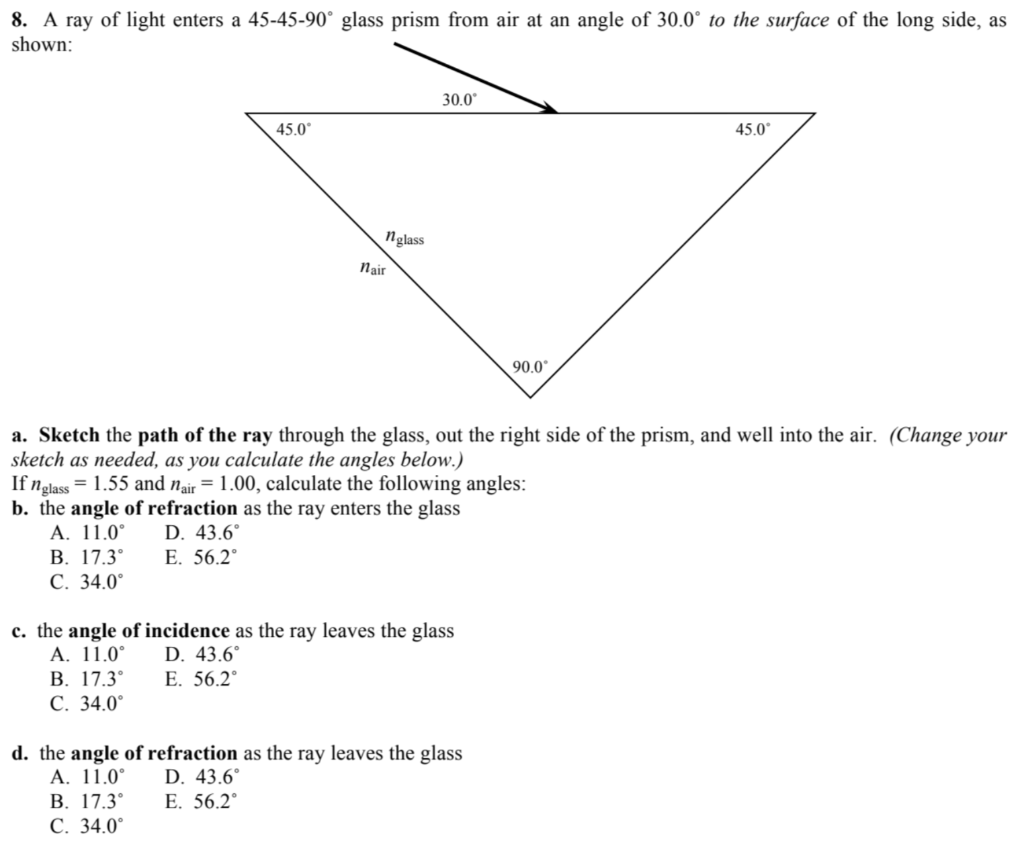8. A ray of light enters a 45-45-90° glass prism from air at an angle of 30.0° to the surface of the long side, as shown: 30.09 45.0° 45.0° nglass nair 900 a. Sketch the path of the ray through the glass, out the right side of the prism, and well into the air. (Change your sketch as needed, as you calculate the angles below.) If n glass = 1.55 and Nair = 1.00, calculate the following angles: b. the...

• ### 1. A beam of white light goes from air into water at an incident angle of...

1. A beam of white light goes from air into water at an incident angle of 67.0°. At what angles are the green (550 nm) and violet (410 nm) parts of the light refracted? (Enter your answer to at least one decimal place.) 2. The index of refraction for orange and violet light in diamond are respectively 2.415 and 2.458. The index of refraction for orange and violet light in fused quartz are respectively 1.456 and 1.468. Based on this...

• ### A beam of light containing red (660 nm) and violet (410 nm) wavelengths travels from air,...

A beam of light containing red (660 nm) and violet (410 nm) wavelengths travels from air, through a flat piece of crown glass 2.54 cm thick, and then back to air. (a) If the beam has an angle of incidence of 18.0° in air, determine the angle at which the two colors of light emerge from the crown glass. The index of refraction respectively for red and violet light in crown glass is 1.512 and 1.530. (Enter a number to...

• ### When a beam of light, originally traveling in air, enters a piece of glass having an...

When a beam of light, originally traveling in air, enters a piece of glass having an index of refraction of 3/2, its frequency A) Increases by a factor of 3/2 B) Is reduced to 2/3 it's original value C) Is unaffected

• ### The index of refraction for a certain type of glass is 1.635 for blue light and 1.607 for red light. When a beam of...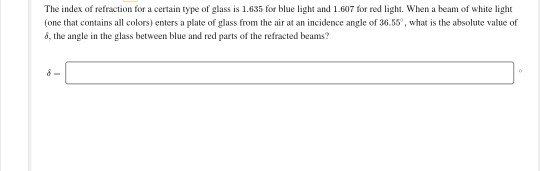The index of refraction for a certain type of glass is 1.635 for blue light and 1.607 for red light. When a beam of white light (one that contains all colors) enters a plate of glass from the air at an incidence angle of 36.55, what is the absolute value of 6, the angle in the glass between blue and red parts of the refracted beams?